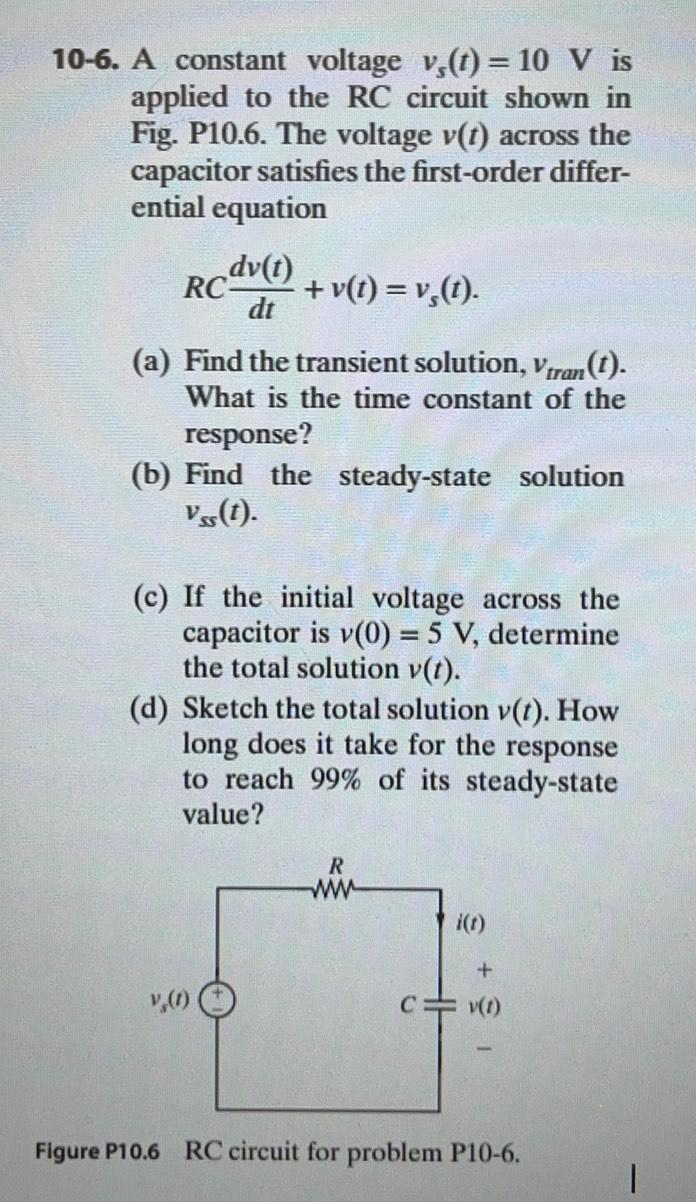Question:

# 10-6. A constant voltage vo(t) = 10 V is applied to the RC circuit shown in Fig. P10.6. The voltage v(t) across the capacitor sa10-6. A constant voltage vo(t) = 10 V is applied to the RC circuit shown in Fig. P10.6. The voltage v(t) across the capacitor satisfies the first-order differ- ential equation dv(t) RC + v(t) = v;(t). dt (a) Find the transient solution, Vtran(t). What is the time constant of the response? (b) Find the steady-state solution vs(t). (c) If the initial voltage across the capacitor is v(0) = 5 V, determine the total solution v(t). (d) Sketch the total solution v(t). How long does it take for the response to reach 99% of its steady-state value? R + 1,(1) CO) Figure P10.6 RC circuit for problem P10-6. 1